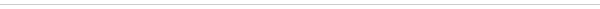## Prayer Times

 table div table+table+table div table{width:100%;padding:0}table div table+table+table div table img{width:96.23%;padding:0;float:none}table div table+table+table div table td{width:100%;padding:0 1.88% 18px}/* styles *//* styles */
 table div table+table+table+table+table div table{width:100%;padding:0}table div table+table+table+table+table div table img{width:96.23%;padding:0;float:none}table div table+table+table+table+table div table td{width:100%;padding:0 1.88% 18px}/* styles */table.module-5{width:75.47%;padding:0}table div table+table+table+table+table+table div table{width:75.47%;float:none;margin-left:auto;margin-right:auto;padding:0}table div table+table+table+table+table+table div table a{border:0 none;text-decoration:none}table div table+table+table+table+table+table div table img{width:100%!important;border:0 none;text-decoration:none}table div table+table+table+table+table+table div table td{width:100%;padding:0}/* styles */
 table div table+table+table+table+table+table+table div table{width:100%;padding:0}table div table+table+table+table+table+table+table div table img{width:96.23%;padding:0;float:none}table div table+table+table+table+table+table+table div table td{width:100%;padding:0 1.88% 18px}/* styles */## Referendum and Election Results

OneCommunityOneFamily
Supervisory Council Announcement: On Sunday November 17th, 2019, the Supervisory Council of the UMJCA held elections of the Central Board, three Synagogue Boards and two referendums. A record number of over 1820 community members voted at the elections. We would like to wholeheartedly thank all the candidates and community members who have participated in this year's record-breaking elections.
UMJCA Referendum Results
2019 UMJCA Election Results

 table div table+table+table+table+table+table+table+table+table div table{width:100%;padding:0}table div table+table+table+table+table+table+table+table+table div table img{width:96.23%;padding:0;float:none}table div table+table+table+table+table+table+table+table+table div table td{width:100%;padding:0 1.88% 18px}/* styles */## Click on the image to Enlarge or Print

 table div table+table+table+table+table+table+table+table+table+table+table div table td,table.module-10{width:100%;padding:0}table div table+table+table+table+table+table+table+table+table+table+table div table{width:100%;float:none;margin-left:auto;margin-right:auto;padding:0}table div table+table+table+table+table+table+table+table+table+table+table div table a{border:0 none;text-decoration:none}table div table+table+table+table+table+table+table+table+table+table+table div table img{width:100%!important;border:0 none;text-decoration:none}/* styles */
 table div table+table+table+table+table+table+table+table+table+table+table+table div table td,table.module-11{width:100%;padding:0}table div table+table+table+table+table+table+table+table+table+table+table+table div table{width:100%;float:none;margin-left:auto;margin-right:auto;padding:0}table div table+table+table+table+table+table+table+table+table+table+table+table div table a{border:0 none;text-decoration:none}table div table+table+table+table+table+table+table+table+table+table+table+table div table img{width:100%!important;border:0 none;text-decoration:none}/* styles */
 table div table+table+table+table+table+table+table+table+table+table+table+table+table div table{width:100%;padding:0}table div table+table+table+table+table+table+table+table+table+table+table+table+table div table img{width:96.23%;padding:0;float:none}table div table+table+table+table+table+table+table+table+table+table+table+table+table div table td{width:100%;padding:0 1.88% 18px}/* styles */table div table+table+table+table+table+table+table+table+table+table+table+table+table+table+table div table{width:100%;padding:0}table div table+table+table+table+table+table+table+table+table+table+table+table+table+table+table div table img{width:96.23%;padding:0;float:none}table div table+table+table+table+table+table+table+table+table+table+table+table+table+table+table div table td{width:100%;padding:0 1.88% 18px}/* styles */table div table+table+table+table+table+table+table+table+table+table+table+table+table+table+table+table+table div table{width:100%;padding:0}table div table+table+table+table+table+table+table+table+table+table+table+table+table+table+table+table+table div table img{width:96.23%;padding:0;float:none}table div table+table+table+table+table+table+table+table+table+table+table+table+table+table+table+table+table div table td{width:100%;padding:0 1.88% 18px}/* styles */# SHIRA NEWS

## Shaare Shalom - Mashadi Jewish Center

▪ Celebrating the Birth of the Newborn Baby Girl, born to Daniela and Robert Namdar
 ▪ Celebrating the Birth of the Newborn Baby Girl, born to Daniela and Robert Namdar

## Ohr Esther - Young Mashadi Jewish Center

▪ Celebrating the Birth of the Newborn Baby Boy, born to Donna and Tal Peri
▪ Celebrating the Birth of the Newborn Baby Boy, born to Jamie and Nima Baratian
▪ Celebrating the Torah Reading by Jake Hakimian, Son of Carly and Elan Hakimian
 ▪ Celebrating the Birth of the Newborn Baby Boy, born to Donna and Tal Peri
 ▪ Celebrating the Birth of the Newborn Baby Boy, born to Jamie and Nima Baratian
 ▪ Celebrating the Torah Reading by Jake Hakimian, Son of Carly and Elan Hakimian

## Shaare Rachamim

▪ Celebrating the Bar-Mitzvah Torah Reading by Levi Enayatian, Son of Ashkan and Avigail Enayatian
▪ Celebrating the Birth of the Newborn Baby Girl, born to Sara and Robert Nowbakht
 ▪ Celebrating the Bar-Mitzvah Torah Reading by Levi Enayatian, Son of Ashkan and Avigail Enayatian
 ▪ Celebrating the Birth of the Newborn Baby Girl, born to Sara and Robert Nowbakht
 table div table+table+table+table+table+table+table+table+table+table+table+table+table+table+table+table+table+table+table+table div table{width:100%;padding:0}table div table+table+table+table+table+table+table+table+table+table+table+table+table+table+table+table+table+table+table+table div table img{width:96.23%;padding:0;float:none}table div table+table+table+table+table+table+table+table+table+table+table+table+table+table+table+table+table+table+table+table div table td{width:100%;padding:0 1.88% 18px}/* styles */